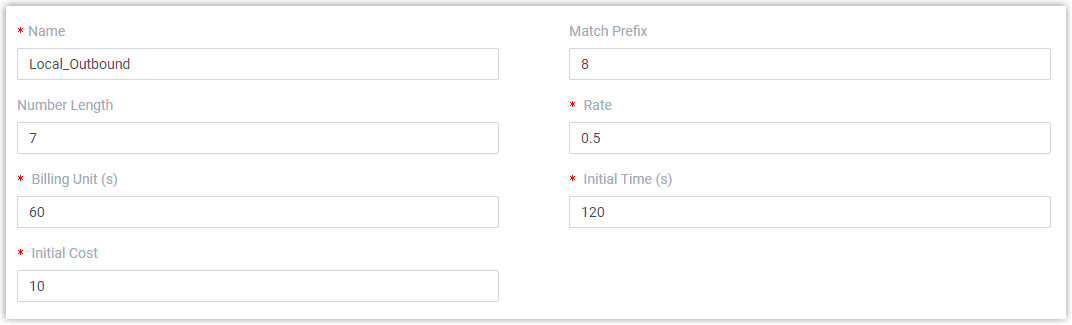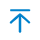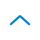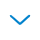# Add a Call Rate Rule

To monitor telecommunication costs for groups and individuals and prevent from potential fraudulent use of resources, you can add a call rate rule for outbound calls, such as local, long-distance, or international calls.

## Restrictions

• A maximum number of 30,000 call rate rules is supported on Yeastar P-Series Software Edition.

## Procedure

1. Log in to PBX web portal, go to Reports and Recordings > Call Reports.
2. Under Rate tab, click Add.
3. Set up a call rate rule:• Name: Enter a name to help you identify the call rate rule. For example, enter Local_Outbound.
• Match Prefix: Optional. Define the prefix of outbound number to match the call rate rule.
Note: The system matches call rate rules based on the outbound number instead of the dialed number.
• If you leave this field blank, then all the outbound numbers will apply this rule.
• If you set a specific value, then only outbound numbers starting with the prefix will apply this rule.
• Number Length: Optional. Define the length of the outbound number to match the call rate rule.
• If you leave this field blank, then all the outbound numbers will apply this rule.
• If you set a specific value, then only the outbound number whose length is shorter than or equal to the value will apply this rule.
• Rate: Enter a call rate. After the initial time, each billing unit will be charged with this rate.
• Billing Unit (s): Define the time increment (in seconds) that will be used to calculate the fee for a call after the initial time. The default value is 60 seconds.

For example, set Rate to 0.5 and Billing Unit to 60 seconds. In this way, the fee for a call will increase by 0.5 every 60 seconds.

• Initial Time (s): Define the initial period of time (in seconds) during which the call will be charged with the initial cost.
• Initial Cost: Define the fixed cost incurred over the preset initial time.

For example, set Initial Time to 120 seconds and Initial Cost to 2. In this case, it costs 2 for the call within 2 minutes. After 2 minutes, the call will be charged with the preset rate.

4. Click Save and Apply.

## Result

• The call rate rule is created. Any outbound calls matching the rule will be charged with the call rate as the following formula from now on.

```Amount = Initial Cost + [(Talk Duration - Initial Time) / Billing Unit] * Rate```

Note: If the value to be multiplied by rate has decimals, it will be rounded upwards to the nearest integer.
• If you create more than one call rate rules in the list, the system will match outbound calls with these rules from the top down. You can clickto adjust the priority.

## What to do next

You can check the call accounting fee for extensions, extension groups, or departments in call reports.

For more information, see Extension Call Accounting Report and Extension Call Accounting Details Report.